# Random variable

(diff) ← Older revision | Latest revision (diff) | Newer revision → (diff)

One of the basic concepts in probability theory. The role of random variables and their expectations (cf. Mathematical expectation) was clearly pointed out by P.L. Chebyshev (1867; see ). The realization that the concept of a random variable is a special case of the general concept of a measurable function came much later. A full exposition, free from any superfluous restrictions, of the basics of probability theory in a measure-theoretical setting was first given by A.N. Kolmogorov (1933; see ). This made it clear that a random variable is nothing but a measurable function on a probability space. This has to be clearly stated, even in an elementary exposition on probability theory. In the academic literature this point of view was adopted by W. Feller (see the foreword to , where the exposition is based on the concept of a space of elementary events, and where it is stressed that only in this context the notion of a random variable becomes meaningful).

Let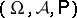be a probability space. A single-valued real-valued function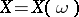defined onis called a random variable if for any realthe set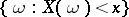belongs to the class. Letbe any random variable and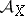the class of subsets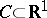for which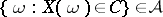; this is a-algebra. The class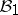of all Borel subsets of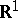is always contained in. The measure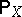defined onby the equation,, is called the probability distribution of. This measure is uniquely determined by the distribution function of:The valuesfor(that is, the values of a measure extendingto) are not, in general, uniquely determined by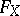(a sufficient condition for uniqueness is so-called perfectness of the measure; see Perfect measure, and also ). This must constantly be borne in mind (for example, when proving that the distribution of a random variable is uniquely determined by its characteristic function).

If a random variabletakes a finite or countable number of pairwise distinct valueswith probabilities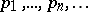(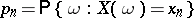), then its probability distribution (which is said to be discrete in this case) is given by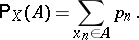The distribution ofis called continuous if there is a function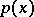(called the probability density) such that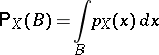for every interval(or equivalently, for every Borel set). In the usual terminology of mathematical analysis this means thatis absolutely continuous with respect to Lebesgue measure on.

Several general properties of the probability distribution of a random variableare sufficiently characterized by a small number of numerical characteristics. For example, the median (in statistics) and quantiles (cf. Quantile) have the advantage that they are defined for all distributions, although the most widely used are the mathematical expectation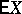and the dispersion (or variance)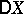of. See also Probability theory.

A complex random variableis determined by a pair of real random variables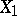andby the formulaAn ordered set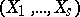of random variables can be regarded as a random vector with values in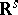.

The notion of a random variable can be generalized to the infinite-dimensional case using the concept of a random element.

It is worth noting that in certain problems of mathematical analysis and number theory it is convenient to regard the functions involved in their formulation as random variables defined on suitable probability spaces (see  for example).

How to Cite This Entry:
Random variable. Encyclopedia of Mathematics. URL: http://encyclopediaofmath.org/index.php?title=Random_variable&oldid=11506
This article was adapted from an original article by Yu.V. Prokhorov (originator), which appeared in Encyclopedia of Mathematics - ISBN 1402006098. See original article